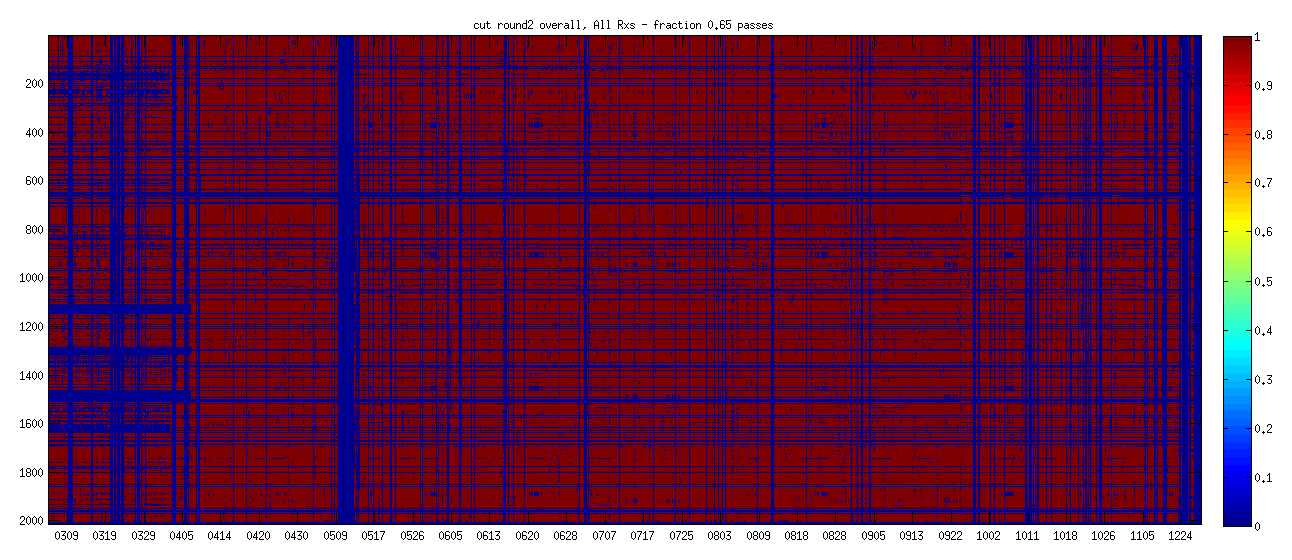## Observing efficiency for BICEP3 and Keck during 2018

### 2020 Oct 19 - Clem Pryke

This posting follows from 20190509_b3_obseff where I took a look at observing efficiency for BICEP3 in 2017 (95GHz). The question was asked as to how this may differ at higher frequencies.

First please take a look at the plots below which show the data passing binary quality cuts for BICEP3 and Keck in 2018. BICEP3 is all 95GHz and Keck is 4/5 220GHz and 1/5 270GHz. On the x-axis is date in MMDD format. On the y-axis is nominally working channel number. Horizontal blue lines are channels which never actually work but didn't get removed from the list. The nominal number of light channels for BICEP3 is 2400 and for Keck it is 2480. The first 4 receivers of Keck are 220GHz and the 5th is 270GHz - from about 1700 on the y-axis onwards - we can see the transition going up the plot between Oct 3 and Oct 13 on the x-axis since bad weather events tend to last longer at 270GHz.

BICEP3 2018:Keck 2018:The map making process records the number of pair-seconds binned into each map pixel. Taking the sum over all pixels for 95/220/270 GHz gives $$7.02\times10^9$$, $$4.06\times10^9$$, $$0.71\times10^9$$ seconds for 95/220/270GHz respectively. The number of light pairs are 1200, 992 and 248 respectively so comparing to the number of pair seconds in a year:

$$(7.02\times10^9)/(1200\times365\times24\times60^2)=0.186$$ at 95GHz

$$(4.06\times10^9)/( 992\times365\times24\times60^2)=0.130$$ at 220GHz

$$(0.71\times10^9)/( 248\times365\times24\times60^2)=0.090$$ at 270GHz.

The above is just the binary cuts. In an ideal world all the pairs being coadded would carry equal weight. In reality some are lower noise than others so the effective efficiency factor is lower than the above. In principle the map making process keeps track of this and I will post about it another time.